# Let's Get Started

We are going to explore how to find the x-intercept and y-intercept using graphs, tables, and equations.  You will determine what each intercept represents.

TEKS Standards and Student Expectations

A(1) Mathematical process standards. The student uses mathematical processes to acquire and demonstrate mathematical understanding. The student is expected to:

A(1)(A) apply mathematics to problems arising in everyday life, society, and the workplace

A(1)(D) communicate mathematical ideas, reasoning, and their implications using multiple representations, including symbols, diagrams, graphs, and language as appropriate

A(3) Linear functions, equations, and inequalities. The student applies the mathematical process standards when using graphs of linear functions, key features, and related transformations to represent in multiple ways and solve, with and without technology, equations, inequalities, and systems of equations. The student is expected to:

A(3)(C)  graph linear functions on the coordinate plane and identify key features, including x-intercept, y-intercept, zeros, and slope, in mathematical and real-world problems

Resource Objective(s)

Given algebraic, tabular, and graphical representations of linear functions, the student will determine the x- and y-intercepts of the function and interpret the meaning of intercepts within the context of the situation.

Essential Questions

How can you determine the x-intercept and y-intercept by looking at a graph?

How can you determine the x-intercept and y-intercept by looking at a table?

How can you determine the x-intercept and y-intercept by looking at an equation?

What do the intercepts tell us about the function?

Vocabulary

# Introduction

Linear functions have the following two important characteristics:

Linear functions can appear in a variety of representations.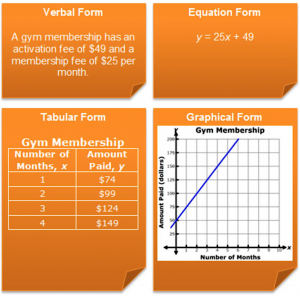Through this exercise, you will investigate different ways to determine and interpret the intercepts of a linear function.

# Determining and Interpreting Intercepts from a GraphAn intercept of any function is a point where the graph of the function crosses, or intercepts, the x-axis or y-axis.

Because there are two axes on a coordinate plane, there can be a x-intercept, which is the point where the graph crosses the x-axis (horizontal axis), or a y-intercept, which is the point where the graph crosses the y-axis (vertical axis) or both.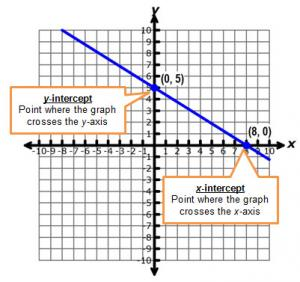When the linear function is used to represent a real-world situation, the intercepts have significant meaning in the context of the problem.

Pause and Reflect

How can you distinguish between the x-intercept and the y-intercept from a graph?

Practice

Interpret both the x- and y-intercepts in the relationships shown in the graphs below.

1. The graph shown below represents the number of tickets that a theater must sell in order to generate \$350 in revenue from ticket sales.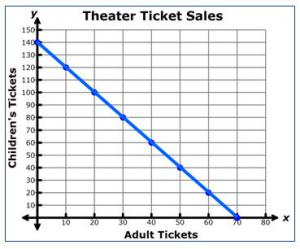2. A hiker is hiking down a canyon toward the canyon floor. On the graph below you can see her height above the canyon floor as a function of time.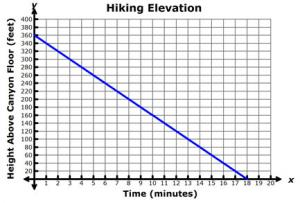# Determining and Interpreting Intercepts from a Table

You can also use a table of values to determine and interpret the intercepts of a function. The graph below represents the relationship between the floor number and the amount of time that Senedra has been in an elevator. Senedra is in an office building in Europe, where the ground floor is labeled "Floor 0" and the next floor up is labeled "Floor 1."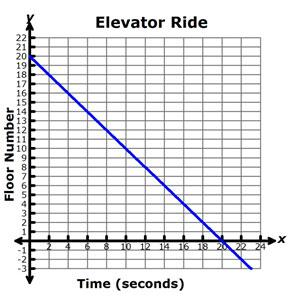Copy and paste the table below into your notes. Use the elevator graph above to complete the table.  Click on the plus sign to check your answers.

• What are the coordinates of the y-intercept?
• What does the y-intercept represent in this situation?
• What are the coordinates of the x-intercept?
• What does the x-intercept represent in this situation?

Practice

Interpret both the x- and y-intercepts in the relationships shown in the tables below.

1. The table below shows the distance remaining in a bus trip from Houston to Dallas as a function of time. How far is it in miles from Houston to Dallas? How many hours will the trip take?

Time in Hours, x Distance in Miles, y
0 240
1 180
2 120
1 1/2 90
4 0

2. A farmer has a field of a certain size in which he or she can decide to plant corn or wheat. The number of bushels of corn or wheat that the farmer can harvest from the field is shown in the table below. How many bushels can be harvested if he or she only plants corn? How many bushels can be harvested if he or she only plants wheat?

Number of Bushels of Corn, x Numer of Bushels of Wheat, y
0 300
40 250
70 212.5
166 92.5
240 0

# Determining and Interpreting Intercepts from an Equation

Linear equations can be written in two forms.

Slope-Intercept Form Standard Form
y = mx + b Ax + By = C

In addition to determining and interpreting intercepts from graphs and tables, you can also determine and interpret intercepts directly from an equation.

To determine an intercept from an equation, simply substitute 0 and solve for the other variable.

x-intercept: (x, 0)
y-intercept: (0, y)

Practice: Slope-Intercept Form

In the problems below, drag the mathematical steps of solving the equation into the correct descending order in each row. Use the completed solutions to look for patterns in how you can determine the x-intercept and y-intercept for a linear equation that is given in slope-intercept form.

Practice: Standard Form

In the problems below, drag the mathematical steps of solving the equation into the correct descending order in each row. Use the completed solutions to look for patterns in how you can determine the x-intercept and y-intercept for a linear equation that is given in standard form.

# Summary

So now you've investigated several different ways to determine the x-intercept and y-intercept of a linear function. You used graphs, tables, and equations to determine the intercepts of linear functions. You also investigated how to interpret the intercepts within the context of a situation.

Consider the graph, equation, and table shown below.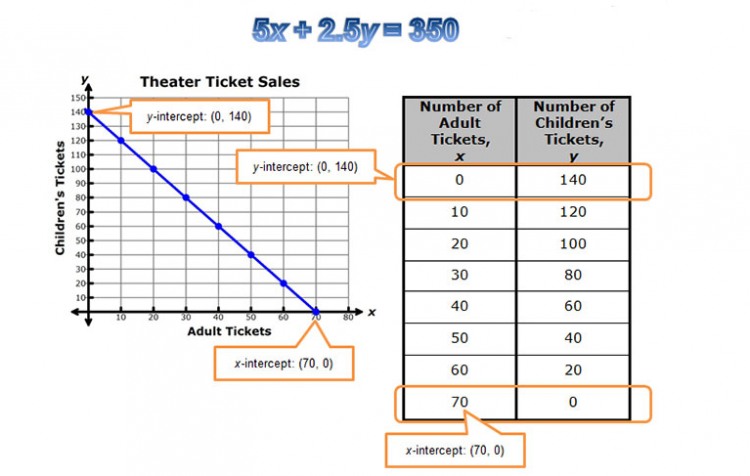In each representation, the x-intercept is the point where the graph of the line crosses the x-axis, or the ordered pair (x, 0). The y-intercept is the point where the graph of the line crosses the y-axis, or the ordered pair (0, y).